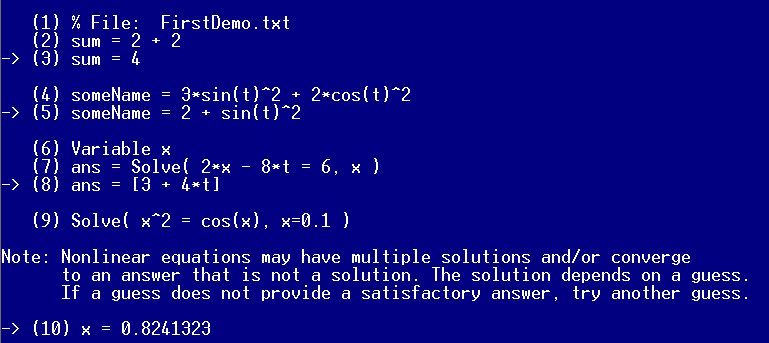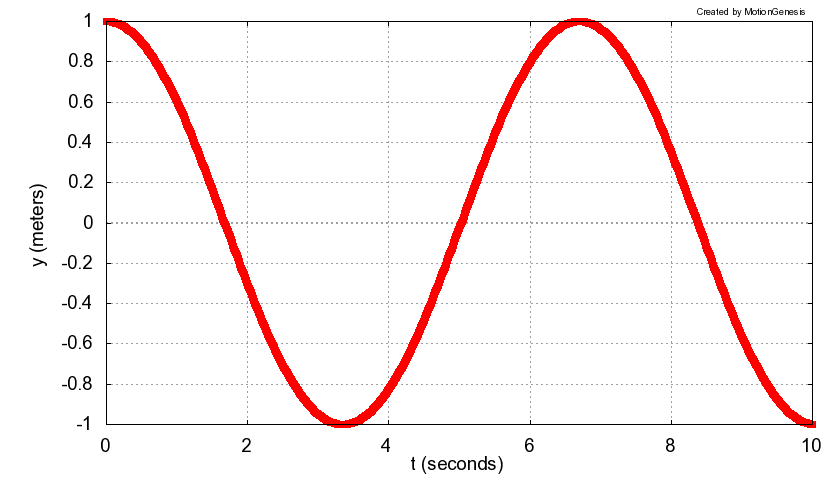Basic input/outputTo invoke the program   (after the program has been installed):
• Double-click on   MotionGenesisStartHere   (or the MotionGenesis executable)
• Or, from the operating system prompt, type   MotionGenesis
On line (1),   type     sum = 2 + 2
Press   Enter   to see the response   -> sum = 4 .
Next, enter a symbolic expression and see the automatic simplification.
```    someName = 3*sin(t)^2 + 2*cos(t)^2
->  someName = 2 + sin(t)^2
```

To solve a linear algebraic equation for x, type:
```   Variable x
ans = Solve( 2*x - 8*t = 6,  x )
```

To solve a nonlinear algebraic equation with a guess for x, type:
```   Solve( x^2 - cos(x) = 0,  x = 0.1 )
```

To solve an ODE (ordinary differential equation), type:
```   Variable    y'' = -sin(y)
Input       y = 1,  y' = 0,  tFinal = 10
OutputPlot  t, y
ODE()       FirstDemo     % Solve the ODE, try to plot.
ODE()       FirstDemo.m   % Solve/plot with MATLAB®.
```

Save input   to the file   FirstDemo.txt,   and exit the program by typing
```   SAVE  FirstDemo.txt
QUIT
```PlotGenesis™ MotionGenesis PlottingEdit   FirstDemo.txt   with a   text-editor   (e.g,, NotePad, SimpleText, TextEdit, Emacs).
For example, put the following comment line at the top of the file (and save the file):
```   %File: FirstDemo.txt
```
Run   the modified text file (MotionGenesis command file)     FirstDemo.txt

Save input and output (input commands together with output responses) to the file   FirstDemo.html,   by typing
```   SAVE  FirstDemo.html
```
To view FirstDemo.html, double-click on the file FirstDemo.html (which should be in your MotionGenesis folder).

For general help and/or a list of commands, type   HELP
For help with a command (e.g., Solve), type   Help SOLVE

Optional: Special symbols
%    Comment delimiter. Characters following the   %   symbol are ignored. % This is a comment.
%%    Inserts comments into   MATLAB®,   C,   or   Fortran codes. %% This comment is written to code.
&    Multi-line continuation symbol.
 longLine = 1 + 2 + 3 + 4 + 5 + 6 + 7 + 8 + 9   & + 9 + 8 + 7 + 6 + 5 + 4 + 3 + 2 + 1
'    Denotes ordinary time-derivative x'   and   x''
>    Last symbol in the name of a vector Fred>
[ ]    Used to designate the element of a matrix Sue[2,3] = 57.4
,   ;    Separates elements and rows in a matrix A = [1, 2, 3; 4, 5, 6]
:    Denotes a range 2:7   denotes   2, 3, 4, 5, 6, 7
:=    Automatically overwrites an expressions without query Keith = 3.8     Keith := 4.2